# Domain and Range of a Function

Go back to  'Functions'

Consider the set A = {1, 2, 3, 4}. Let us define a function $$f\left( x \right) ={x^2}$$ with the input set as the set A. Let us name the output set as set B. We thus have the following scenario: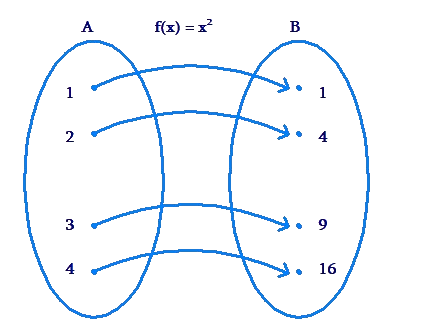The set A consists of all the input values, while the set B consists of all the output values. We now define the following two terms:

• Domain of a function – this is the set of input values for the function. In the example above, the domain of $$f\left( x \right)$$ is set A.

• Range of a function – this is the set of output values generated by the function (based on the input values from the domain set). In the example above, the range of $$f\left( x \right)$$ is set B.

Let’s take another example. Let X be the set {$$- 1$$ , 0, 1, 2}, while $$g\left( x \right)$$ be a function defined as $$g\left( x \right) = {x^3}$$. If we apply the function g on set X, we have the following picture: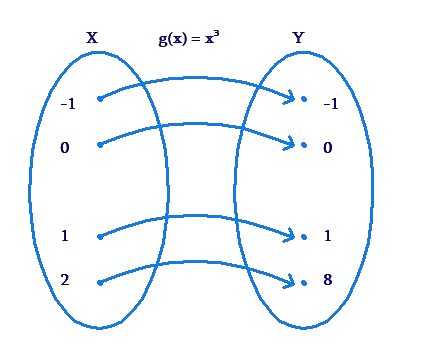The set X is the domain of $$g\left( x \right)$$ in this case, whereas the set Y = {$$- 1$$, 0, 1, 8} is the range of the function corresponding to this domain.

When a function f has a domain as a set X, we state this fact as follows: f is defined on X.

Example 1: Let f be a function defined on $$\mathbb{Z}$$ (the set of all integers), such that $$f\left( x\right) = {x^2}$$. Find the domain and the range of f.

Solution: The domain of f has already been stated in the question: the set of all integers, $$\mathbb{Z}$$ . Now, any integer when squared will generated a positive perfect square. Thus, the set of output values will be these.

${0, 1, 4, 9, 16, …}$

We can thus say that the range is the set of all positive perfect squares. We can write this as follows:

$R = {{n^2},\, n \;in\; \mathbb{Z} }$

Note that since the domain is discrete, the range is also discrete.

Example 2: The plot of a function f is shown below: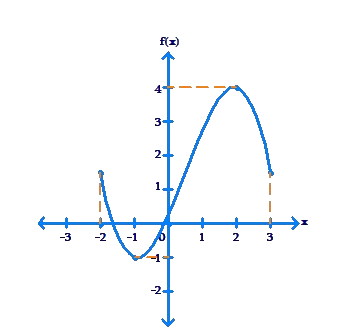Find the domain and range of the function.

Solution: We observe that the graph corresponds to a continuous set of input values, from $$- 2$$ to 3. Thus, the domain of the function is $$\left[ { - 2,3} \right]$$.Also, the variation in the function output is in the continuous interval from $$- 1$$ to 4. Thus, the range of the function is $$\left[ { - 1,4} \right]$$.

Example 3: Let f be a function defined on $$\left[ {- 1,3} \right]$$ such that $$f\left( x\right) = 2x - 1$$. Plot the graph of f and determine its domain and range.

Solution: The graph of f will be linear, as shown below: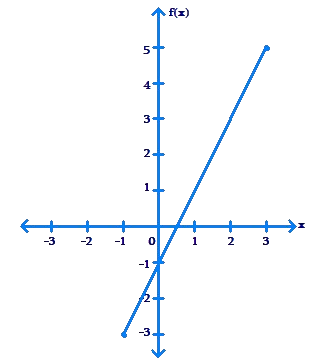The domain is clearly $$\left[ { - 1,3} \right]$$. Also, we note that the function takes all values in the continuous interval from $$- 3$$ to 5. Thus, the range of the function is $$\left[ { - 3,5} \right]$$.

Example 4: f is a function defined on $$\left[ { -2,1} \right]$$ such that $$f\left( x\right) = \frac{1}{2}{x^2}$$. Plot the graph of f, and find its domain and range.

Solution: First, we determine a few markers to aid us in our plotting process:

 x $$- 2$$ $$- 1$$ 0 $$\frac{1}{2}$$ 1 $$f\left( x \right)$$ 2 $$\frac{1}{2}$$ 0 $$\frac{1}{8}$$ $$\frac{1}{2}$$ Point $$\left( { - 2,2} \right)$$ $$\left( { - 1,\frac{1}{2}} \right)$$ $$\left( {0,0} \right)$$ $$\left( {\frac{1}{2},\frac{1}{8}} \right)$$ $$\left( {1,\frac{1}{2}} \right)$$

Using these markers, the plot of has been drawn below: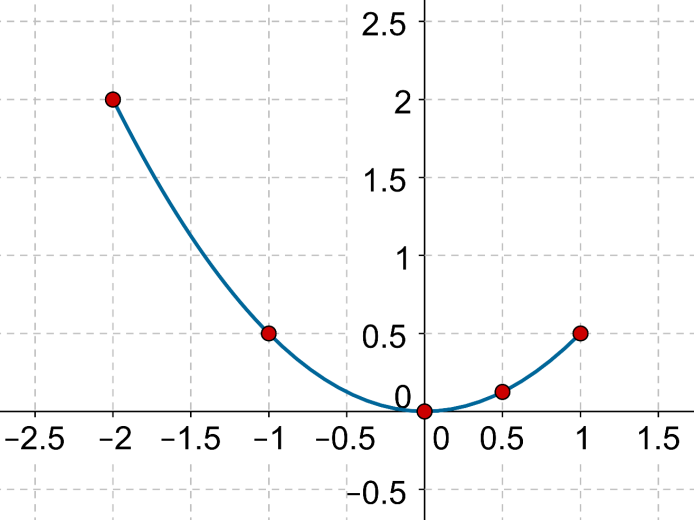The domain of f is clearly $$\left[ { - 2,1} \right]$$. From the plot, it is clear that the range is $$\left[ {0,2}\right]$$.

Example 5: What will be the range of the function $$f\left(x \right) = 1 + {x^2}$$ if the domain is the set of all real numbers?

Solution: If x varies over all real numbers, then $${x^2}$$ takes all values in the set $$\left[ {0,\infty } \right)$$,because $${x^2} \ge 0$$. Thus, $$1 + {x^2}$$ takes all values in the set $$\left[ {1,\infty } \right)$$. This means that the range of f is $$\left[ {1,\infty }\right)$$. This is clear from the following figure, which shows the graph of $$f\left( x \right)$$. Note the variation in output values – from a minimum of 1 towards infinity: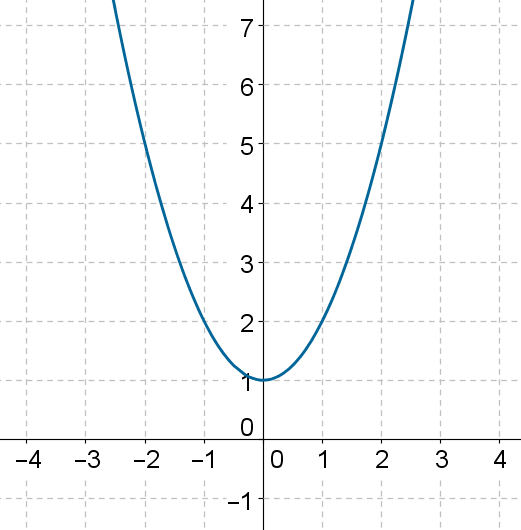Example 6: The function $$f\left( x \right) = 2 + {x^3}$$is defined on a set X, and its range is Y = {$$- 6$$, 1, 2}. What is the domain of the function?

Solution: If $$f\left( x \right) = - 6$$, then $$2 + {x^3} = - 6$$, which means that $$x = - 2$$. Similarly, when $$f\left( x \right) = 1$$, then $$x = - 1$$, and when $$f\left( x \right) = 2$$, then $$x = 0$$. We have the following map: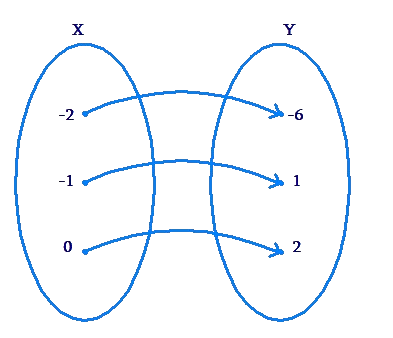Thus, the domain of the function is

$X = {\,( - 2), ( -1), 0}$

## What defines a function?

• A technical definition of a function is: a relation from a set of inputs to a set of possible outputs where each input is related to exactly one output.

## Can range be negative?

• If your set includes negative numbers, the range will still be positive because subtracting a negative is the same as adding. When dealing with range, imagine the numbers on the number line.

## What is a function in algebra?

• An algebraic function is an equation that allows one to input a domain, or x-value and perform mathematical calculations to get an output, which is the range, or y-value, that is specific for that particular x-value. There is a one in/one out relationship between the domain and range.

## How do we find the range of a function?

• The range of a function is the set of all possible values it can produce. No matter what value we give to x, the function is always positive: If x is 2, then the function returns x squared or 4.

## How do you graph a function?

• Graphs of functions are graphs of equations that have been solved for y. It is easy to generate points on the graph. Choose a value for the first coordinate, then evaluate f at that number to find the second coordinate.
Functions
Functions
grade 10 | Questions Set 2
Functions
Functions
grade 10 | Questions Set 1